# 图形相关基础函数

## 使用图形

# 创建一个 1.jpg 的文件
jpeg('1.jpg')
# 绑定trees数据
attach(trees)
# 根据Height和Volume绘制点图形
plot(Height,Volume)
# 自定义图形标题
title("这是一个标题")
# 解除绑定trees数据
detach(trees)
# 返回输出结果
dev.off()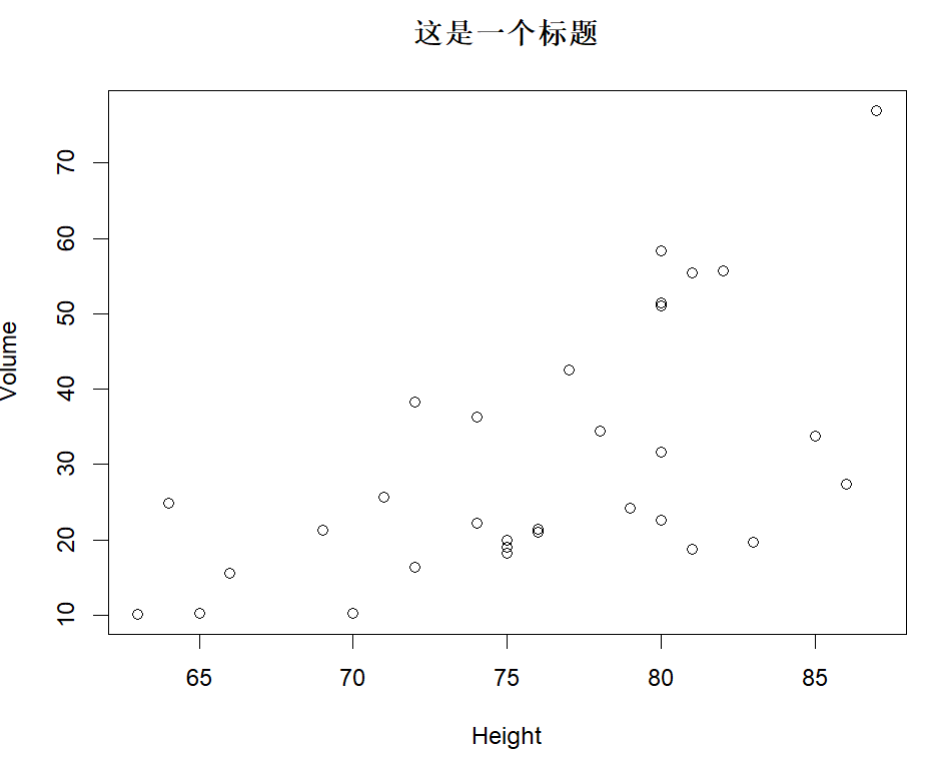## 图形输出

pdf('filename.pdf')输出PDF文件
png('filename.png')输出PNG文件
jpeg('filename.jpg/jpeg')输出JPG文件
bmp('filename.bmp')输出BMP文件

# 图形参数

plot(x, y = NULL, type = "p",  xlim = NULL, ylim = NULL,
log = "", main = NULL, sub = NULL, xlab = NULL, ylab = NULL,
ann = par("ann"), axes = TRUE, frame.plot = axes,
panel.first = NULL, panel.last = NULL, asp = NA,
xgap.axis = NA, ygap.axis = NA,
...)

• x：即x轴数据
• y：即y轴数据

## 符号和线条

pch指定绘制点时使用的符号
cex指定符号的大小。cex是数值型，默认大小为1，2表示放大2倍
lty指定线条类型
lwd指定线条宽度。默认值为1，同cex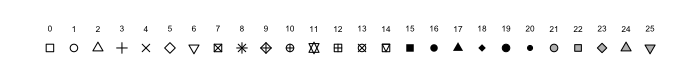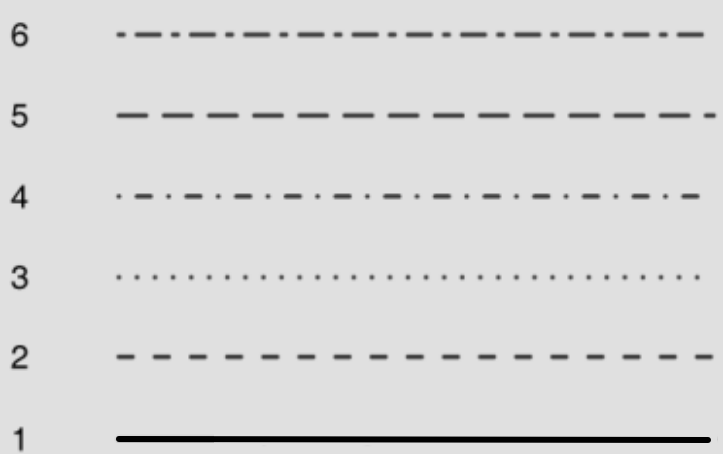## 颜色

col设置绘图颜色。某些函数可以接受颜色值向量并自动循环使用。例如：col=c('red','green)则绘制的图线先红色后绿色，周而复始.
col.axis坐标轴刻度文字的颜色
col.lab坐标轴标签的颜色
col.main标题颜色
col.sub副标题颜色
fg前景色（即图表框的颜色）
bg背景色

col=1
col='white'
col='#FFFFFF'
col=rgb(1,1,1)
col=hsv(0,0,1)


## 文本属性

cex表示绘图大小，默认为1
cex.axis坐标轴刻度字体的大小，大小设置同上
cex.lab坐标轴标签的大小，大小设置同上
cex.main标题的大小，大小设置同上
cex.sub副标题的大小，大小设置同上
---------------------------------------------------
font接收整型参数，用于指定绘图字体样式。（1=常规，2=粗体，3=斜体，4=粗斜体，5=符号字体）
font.axis坐标轴刻度字体样式，同上
font.lab坐标轴标签字体样式，同上
font.main标题字体样式，同上
font.sub副标题字体样式，同上
ps字体磅值（1磅 $\approx$ $\frac{1}{72}$ 英寸），文本最终大小=$ps \times cex$
family绘制文本时使用的字体族。

# 添加文本，自定义坐标轴和图例

## 图形尺寸于边界尺寸

pin以英寸表示图形的尺寸（宽和高）
mai以数值向量表示边界的大小，顺序是下，左，上，右，单位为英寸
mar以数值向量表示边界大小，顺序同上，单位是英分

1英寸=2.54厘米，1英分= $\frac{1}{12}$ 英寸

## 标题

title(main = NULL, sub = NULL, xlab = NULL, ylab = NULL,
line = NA, outer = FALSE, ...)


NA是Not Available的缩写，是一种占位符，可以理解为值=NA，但是不存在

title(main = '这是主标题',sub = '这是副标题',xlab = '这是横坐标标签',ylab = '这是纵坐标标签')


## 坐标轴

axis(side, at = NULL, labels = TRUE, tick = TRUE, line = NA,
pos = NA, outer = FALSE, font = NA, lty = "solid",
lwd = 1, lwd.ticks = lwd, col = NULL, col.ticks = NULL,


side整型，表示在图形哪边绘制坐标轴（1=下，2=左，3=上，4=右）
at数值型向量，表示需要绘制刻度线的位置
labels字符型向量，表示置于刻度线旁边的文字标签（如果为NULL则直接使用at中的值）
pos坐标轴线绘制位置的坐标（即与另一条坐标轴相交的位置）
lty线条类型
col线条和刻度线的颜色
las标签是否平行(=0)或者垂直于(=2)坐标轴
tck刻度线的长度，以相对于绘图区域大小的分数表示（负值表示在图形外侧，正值表示在图形内侧，0表示禁用刻度，1表示绘制网格线）；默认值为 $-0.01$

• 参数axes=FLASE将禁用全部坐标轴（包括坐标轴框架线）
• 参数xaxt='n'yaxt='n'将分别禁用 X 轴或者 Y 轴（会留下框架线，只是去除了刻度）

# 定义数据
x <- c(1:10)
y <- x
z <- 10/x

# 设定图形边界
par(mar=c(5,4,4,8)+0.1)

# 绘制红色直线
plot(x,y,type = 'b',pch=21,col='red',yaxt='n',lty=3,ann=FALSE)

# 绘制蓝色曲线
lines(x,z,type='b',pch=22,col='blue',lty=2)

# 绘制左侧Y轴刻度线
axis(2,at=x,labels = x,col.axis='red',las=2)

# 绘制右侧Y轴刻度线
axis(4,at=z,labels = round(z,digits = 2),col.axis='blue',las=2
,cex.axis=0.7,tck=-.01)

# 添加右侧标题
mtext('y=1/x',side=4,line=3,cex.lab=1,las=2,col='blue')

# 添加横纵坐标标题
title('这是一个样图',xlab = '这是横坐标',ylab = '这是纵坐标',line = 2)

par(opar)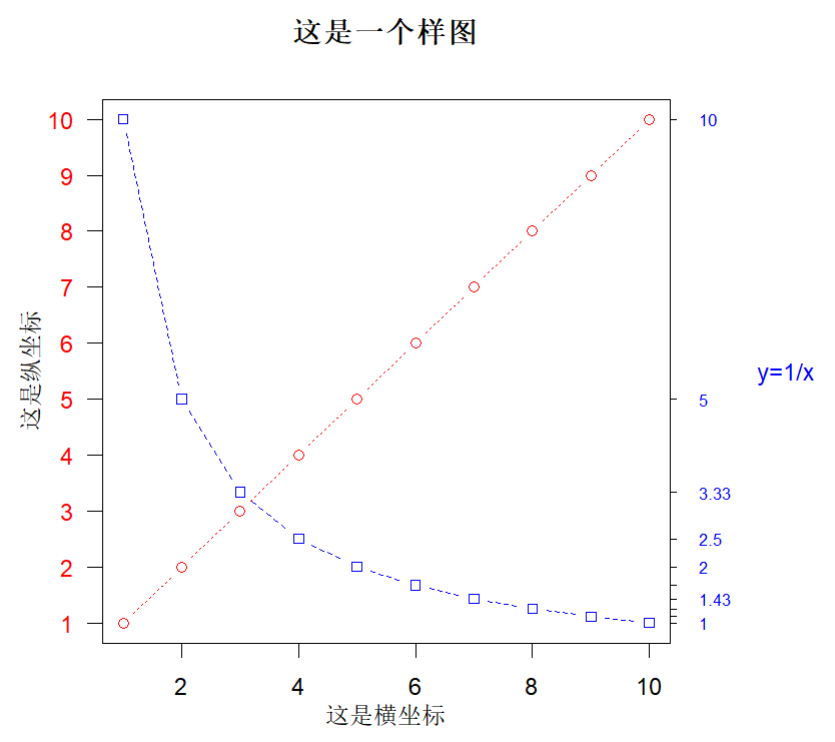line(x, y, iter = 1)


type='p'表示绘制点
type='l'表示绘制线
type='b'表示绘制点和线
type='c'表示曲线连接的空点
type='s'或者type='S'表示阶梯状线
type='h'表示直方图状垂直线
type='n'表示不产生任何点或者线

round()函数是进行四舍五入的函数，函数原型如下：

round(x, digits = 0)

• x：需要处理的数据
• digits：要使用的小数位数或者有效数字的数目

mtext函数是将文本写在图形区域的是个边距的周围。函数原型如下：

mtext(text, side = 3, line = 0, outer = FALSE, at = NA,
adj = NA, padj = NA, cex = NA, col = NA, font = NA, ...)


text标题内容
side文本在坐标轴边框的位置（1=下，2=左，3=上，4=右）
line距离坐标轴边框的距离，值越大越远
las文字是否平行(=0)或者垂直于(=2)坐标轴

## 次要刻度线

minor.tick(nx=2, ny=2, tick.ratio=0.5, x.args = list(), y.args = list())

• nx：指在X轴分割的区域，2即次刻度线将每个区间分为两个区域
• ny：指在Y轴分割的区域，同上
• tick.ratio：表示次刻度相对于主刻度线的大小比例

## 参考线

abline(a = NULL, b = NULL, h = NULL, v = NULL, reg = NULL,
coef = NULL, untf = FALSE, ...)

• h：Y 轴的参考线
• v：X 轴的参考线

# 在垂直 3 坐标和水平 2 坐标，用虚线话画出红色的参考线
abline(h=2,v=3,lty=2,col='red')


## 图例

legend(x, y = NULL, legend, fill = NULL, col = par("col"),
border = "black", lty, lwd, pch,
angle = 45, density = NULL, bty = "o", bg = par("bg"),
box.lwd = par("lwd"), box.lty = par("lty"), box.col = par("fg"),
pt.bg = NA, cex = 1, pt.cex = cex, pt.lwd = lwd,
xjust = 0, yjust = 1, x.intersp = 1, y.intersp = 1,
adj = c(0, 0.5), text.width = NULL, text.col = par("col"),
text.font = NULL, merge = do.lines && has.pch, trace = FALSE,
plot = TRUE, ncol = 1, horiz = FALSE, title = NULL,
inset = 0, xpd, title.col = text.col, title.adj = 0.5,
title.cex = cex, title.font = text.font,
seg.len = 2)


location有两种定位图例位置的方法，第一种是通过指定 X，Y 的值来指定图例的位置（以图例左上角为顶点），第二种方式是通过指定关键字，"bottomright", "bottom", "bottomleft", "left", "topleft", "top", "topright", "right" and "center"，如果使用关键字指定位置，可以通过inset参数来调整其距离边框的位置。
title图例的标题

legend("topleft",title = '图例标题',inset = 0.2,c('A','B'),pch=c(2,5))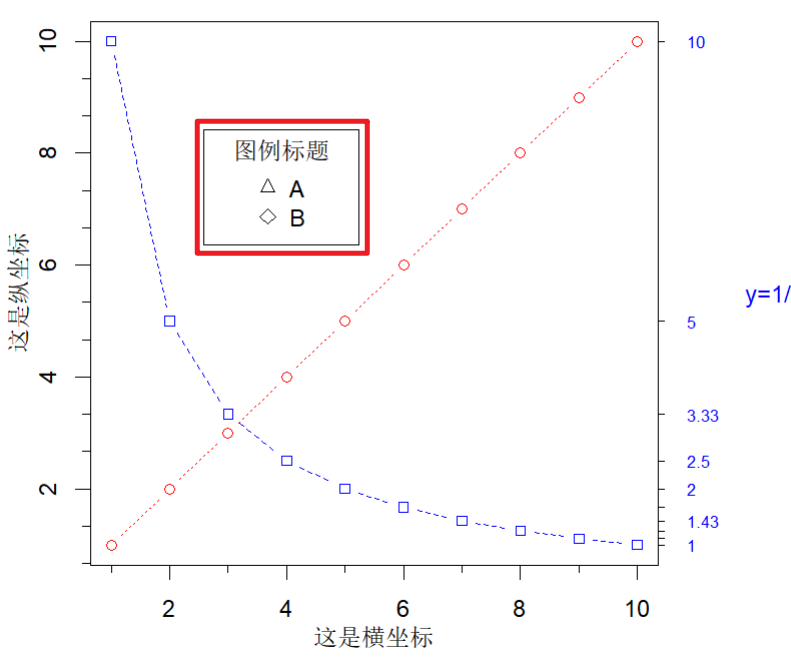## 文本标注

# mtext
mtext(text, side = 3, line = 0, outer = FALSE, at = NA,
adj = NA, padj = NA, cex = NA, col = NA, font = NA, ...)

# text
text(x, ...)


mtext函数已经在坐标轴部分说明了，text的相关参数说明如下;

• x：指定 X 轴的位置
• y：指定 Y 轴的位置
• label：标注内容
• pos：标准内容和点的偏移量

# 创建数据
a <- c(1:10)
b <- 1/c(1:10)

# 绘制图形
plot(a,b)

# 添加坐标的文字
text(1,1,'这是第一个数据',pos=4)
text(2,1/2,'这是第二个数据',pos=4)
text(3,1/3,'这是第三个数据',pos=4)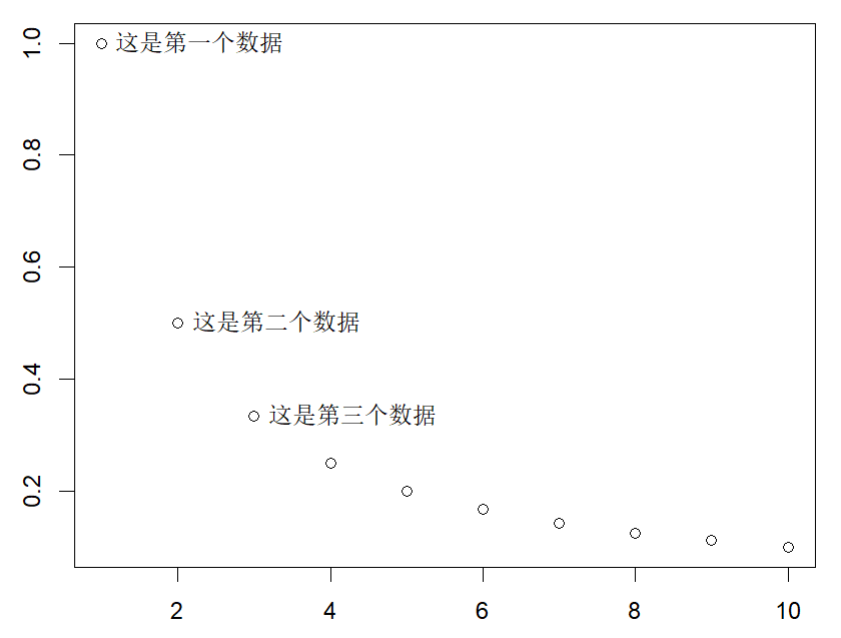### 数学标注

# 使用 expression() 来呈现数学公式
text(2,1/2,labels=expression(frac(1,2)),pos=4)


# 图形的组合

# 绑定数据源
attach(trees)
# 获取初始图形参数
# 设置两行两列
par(mfrow=c(2,2))
# 绘制图形
plot(Height,Volume,main='第一个图')
plot(Height,Girth,main='第二个图')
hist(Height,main='第三个图')
boxplot(Height,main='第四个图')
# 设置图形参数
par(opar)
# 解绑数据源
detach(trees)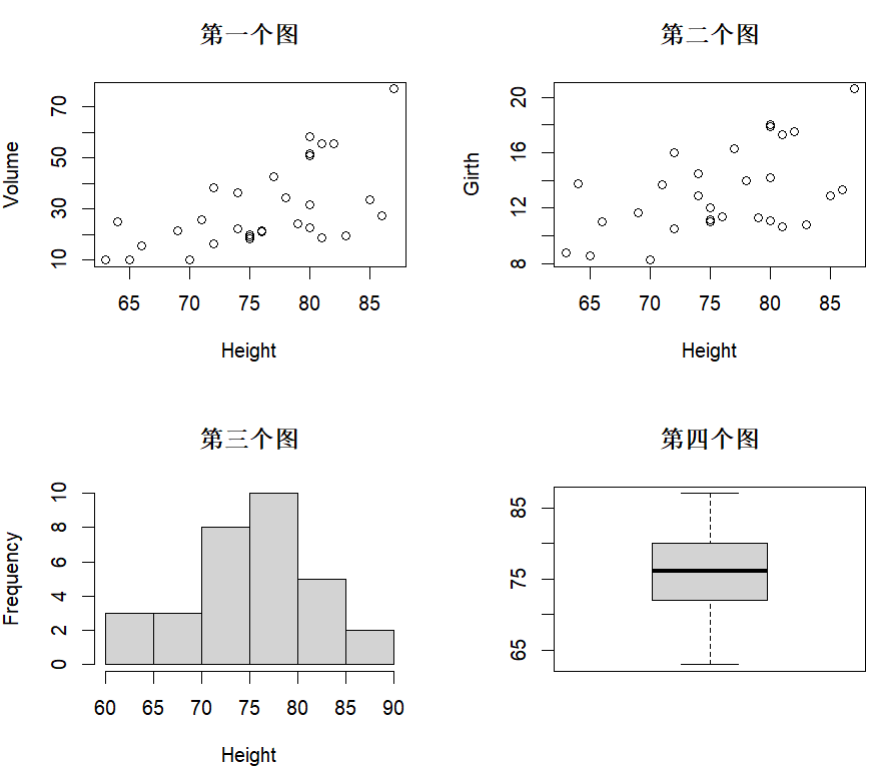layout(mat, widths = rep.int(1, ncol(mat)),
heights = rep.int(1, nrow(mat)), respect = FALSE)

• mat：矩阵类型参数，四个参数表示四个位置

attach(trees)
layout(matrix(c(1,1,2,3),2,2,byrow = TRUE))
hist(Height)
hist(Volume)
hist(Girth)
detach(trees)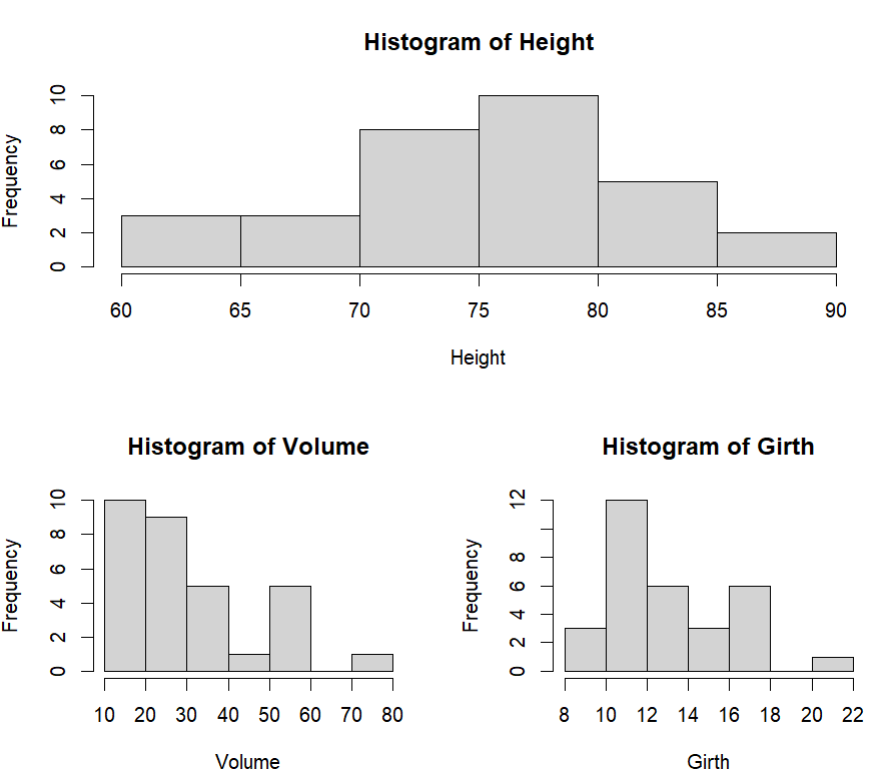• widthsheights：两个函数都接收一个向量参数，是一种比例

attach(trees)
layout(matrix(c(1,1,2,3),2,2,byrow = TRUE)
,widths = c(3,1),heights = c(1,2))
hist(Height)
hist(Volume)
hist(Girth)
detach(trees)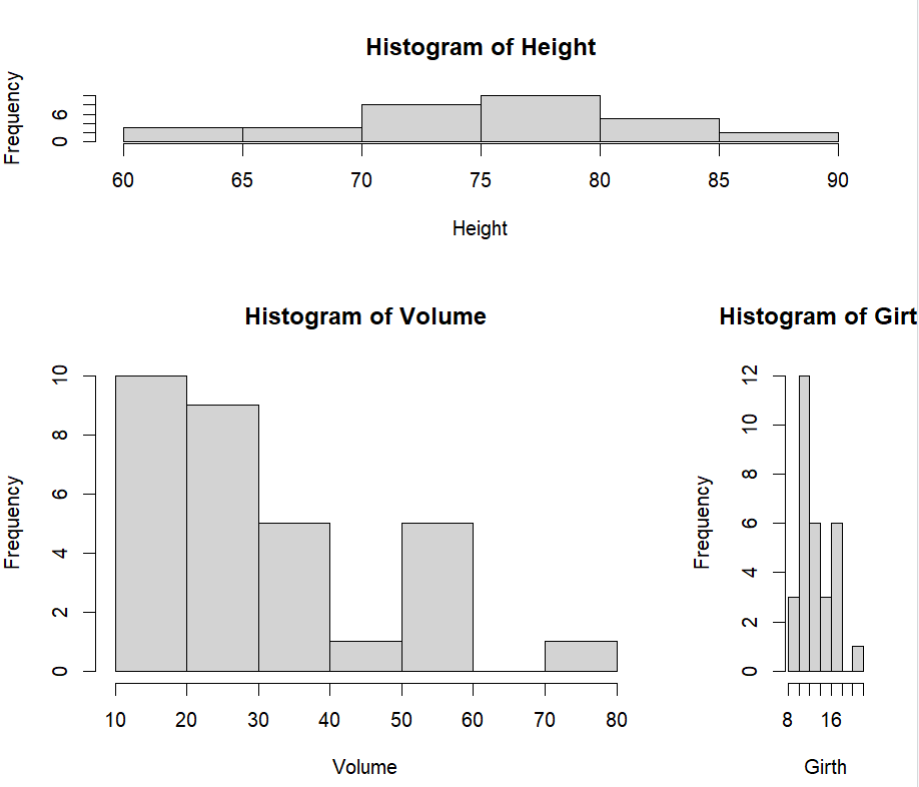## 图形的精细化控制

attach(trees)
par(fig=c(0,0.8,0,0.8))
plot(Height,Volume)
par(fig=c(0,0.8,0.5,1),new=TRUE)
boxplot(Height,horizontal = TRUE,axes=FALSE)
par(fig=c(0.65,1,0,0.8),new=TRUE)
boxplot(Volume,axes=FALSE)
detach(trees)

• new=TRUE：意思是绘制图形的时候不清楚原图像，而是继续叠加绘图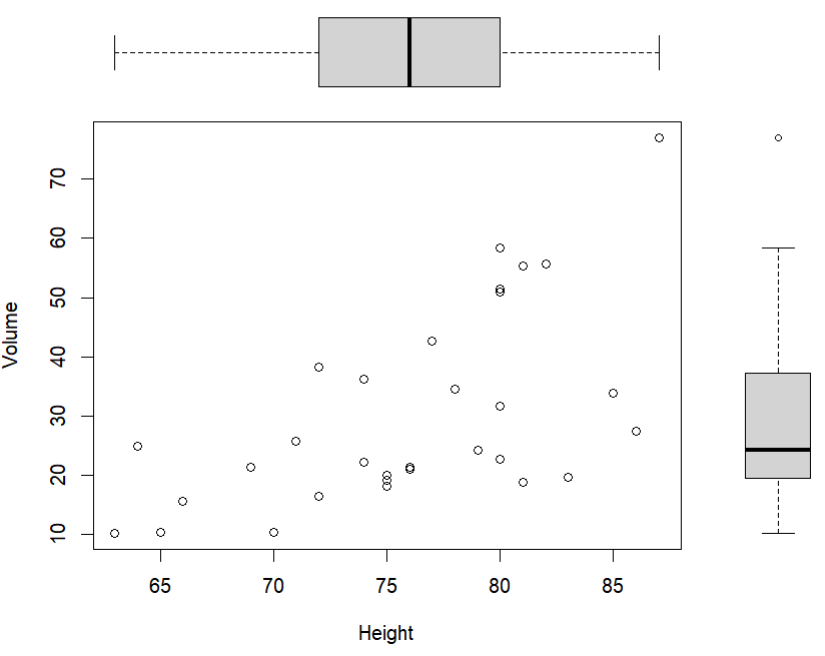fig参数接收一个四参数的向量，它表示的是相对位置，其参数类型为fig=c(x1,x2,y1,y2)，代码par(fig=c(0,0.8,0,0.8))的意思是规定图形的绘制范围为：横向从 0 到 0.8 这个比例范围内，纵向 0 到 0.8 这个比例范围内。

# End

Q.E.D.

••